Ex 4.1

Chapter 4 Class 11 Mathematical Induction
Serial order wise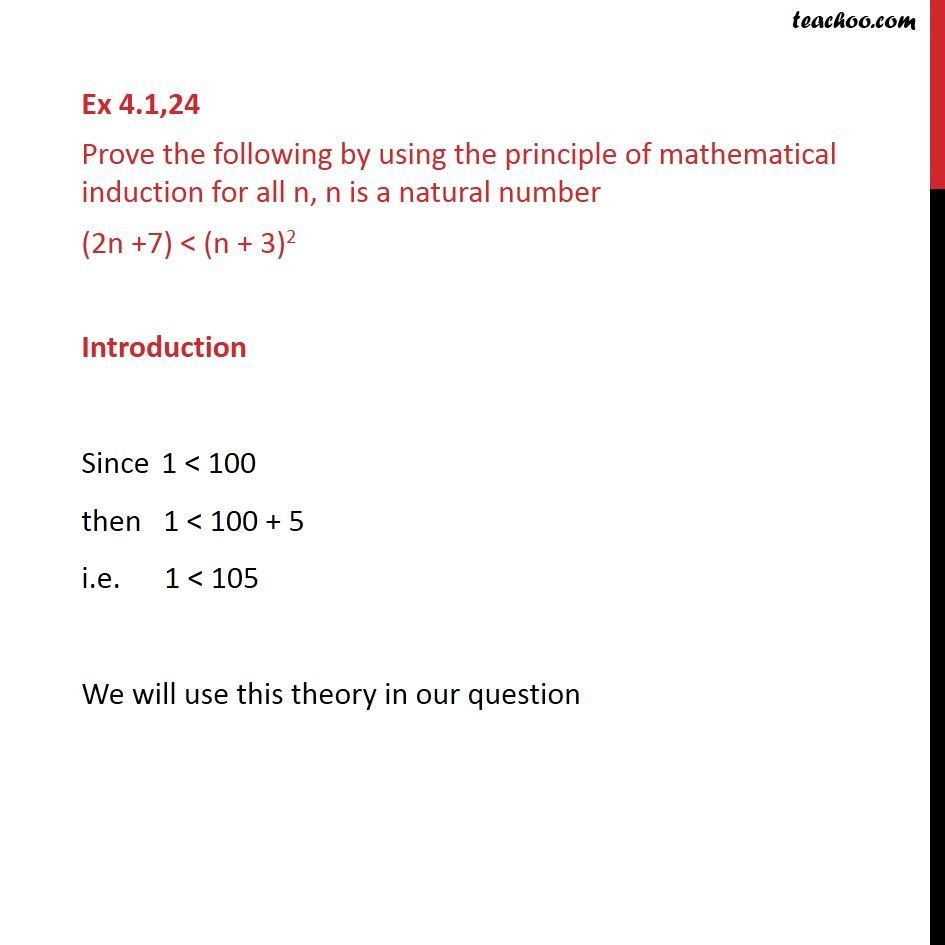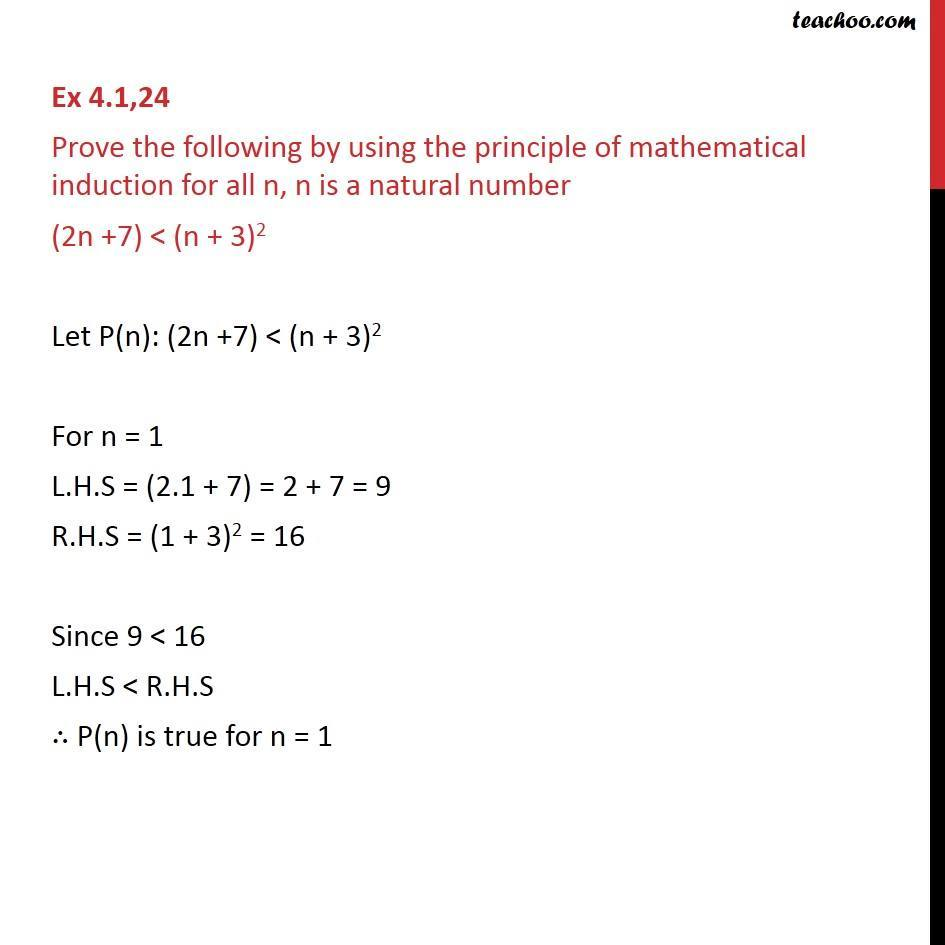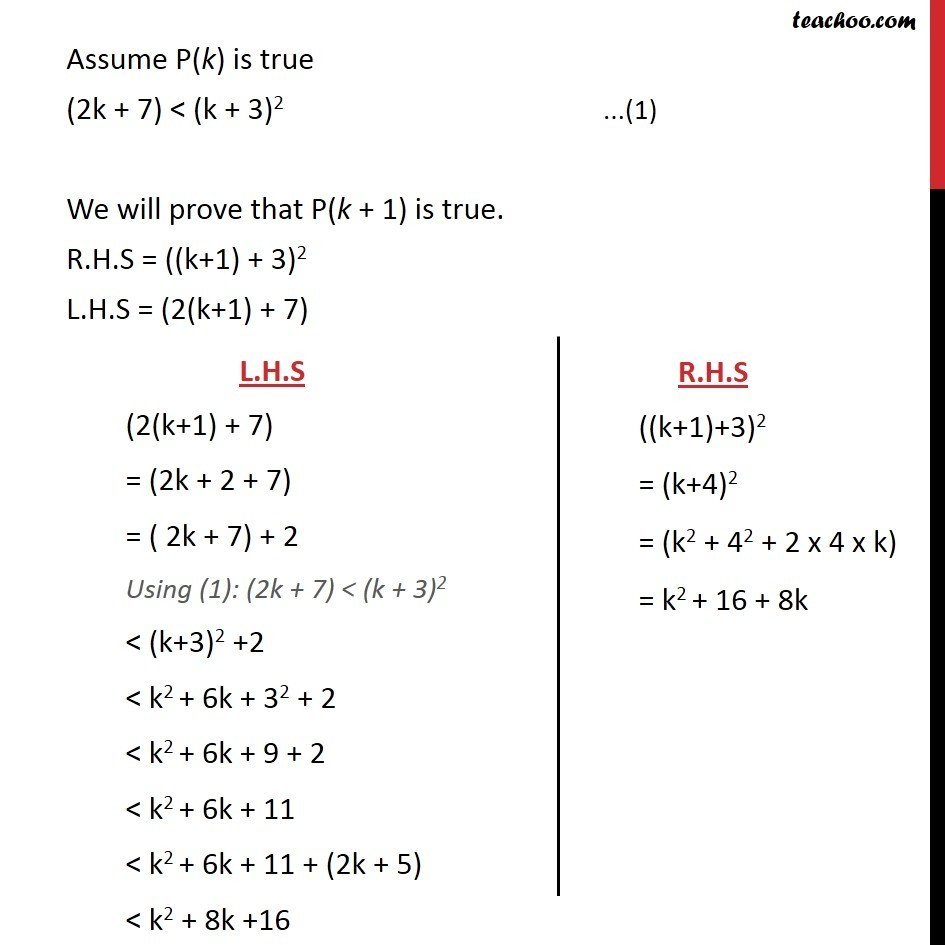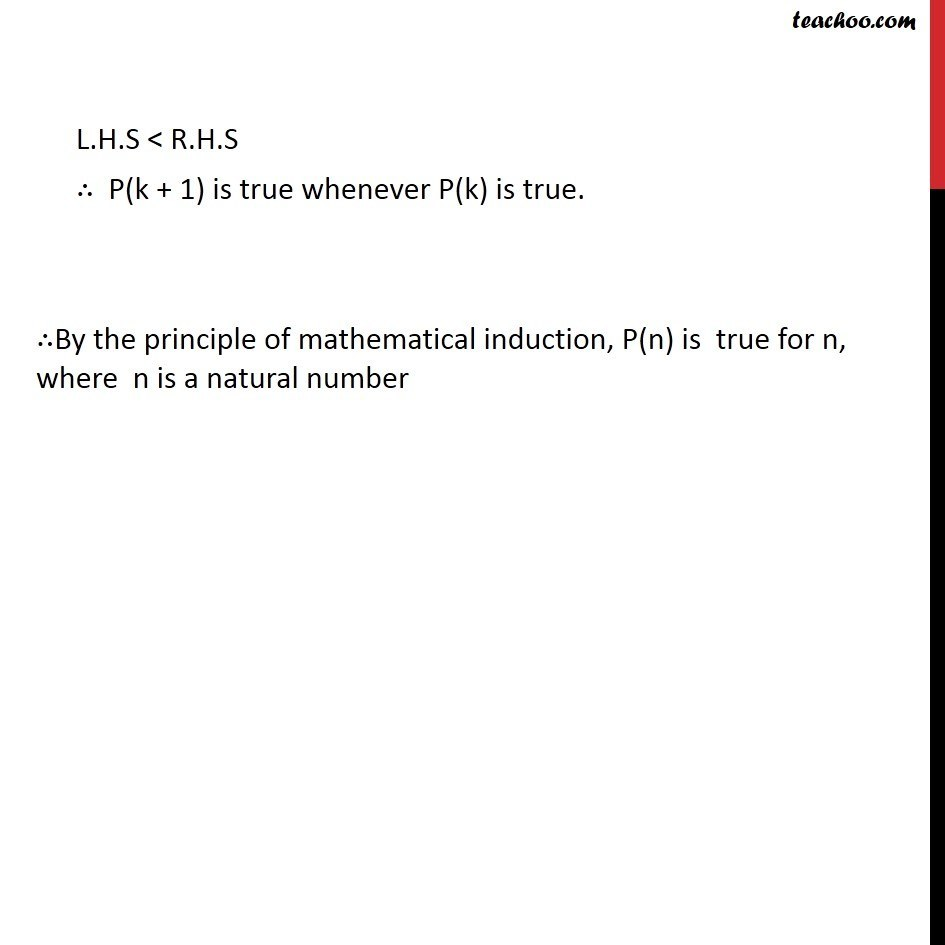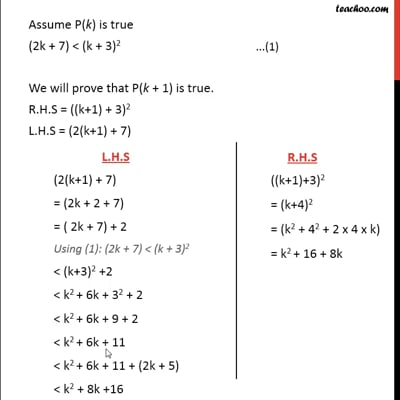This video is only available for Teachoo black users

Solve all your doubts with Teachoo Black (new monthly pack available now!)

### Transcript

Ex 4.1,24 Prove the following by using the principle of mathematical induction for all n, n is a natural number (2n +7) < (n + 3)2 Introduction Since 1 < 100 then 1 < 100 + 5 i.e. 1 < 105 We will use this theory in our question Ex 4.1,24 Prove the following by using the principle of mathematical induction for all n, n is a natural number (2n +7) < (n + 3)2 Let P(n): (2n +7) < (n + 3)2 For n = 1 L.H.S = (2.1 + 7) = 2 + 7 = 9 R.H.S = (1 + 3)2 = 16 Since 9 < 16 L.H.S < R.H.S P(n) is true for n = 1 Assume P(k) is true (2k + 7) < (k + 3)2 We will prove that P(k + 1) is true. R.H.S = ((k+1) + 3)2 L.H.S = (2(k+1) + 7) L.H.S < R.H.S P(k + 1) is true whenever P(k) is true. By the principle of mathematical induction, P(n) is true for n, where n is a natural number